Question

# The powers of two light-bulbs are 60 W and 125 W when connected in parallel across...

The powers of two light-bulbs are 60 W and 125 W when connected in parallel across a 130-V source. What is the power of the bulb that will output less power when the two light-bulbs are connected in series with the same source?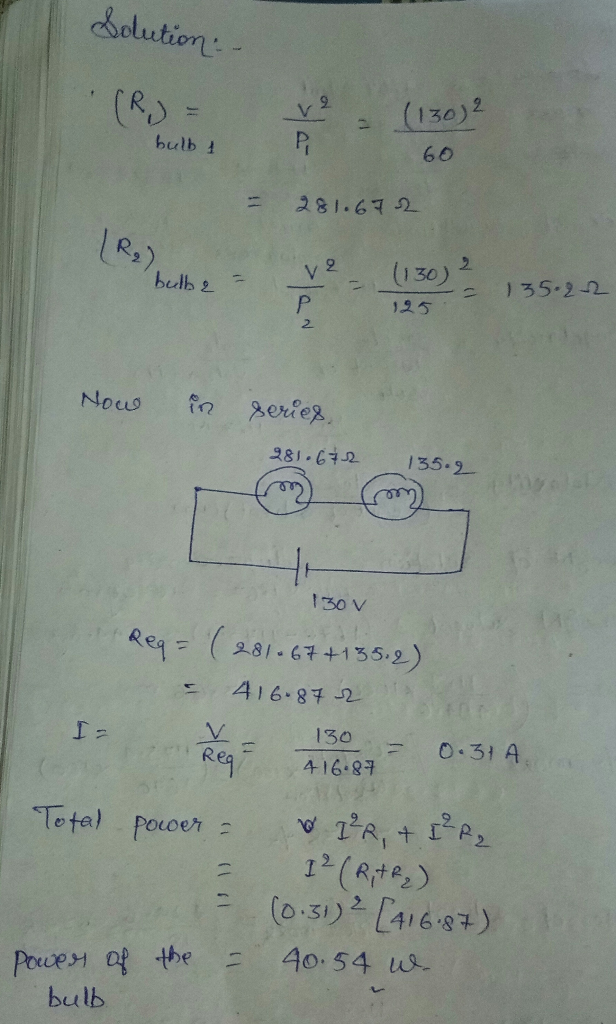#### Earn Coins

Coins can be redeemed for fabulous gifts.

Similar Homework Help Questions
• ### Two light bulbs have resistances of 40 2 and 802. The two light bulbs are now...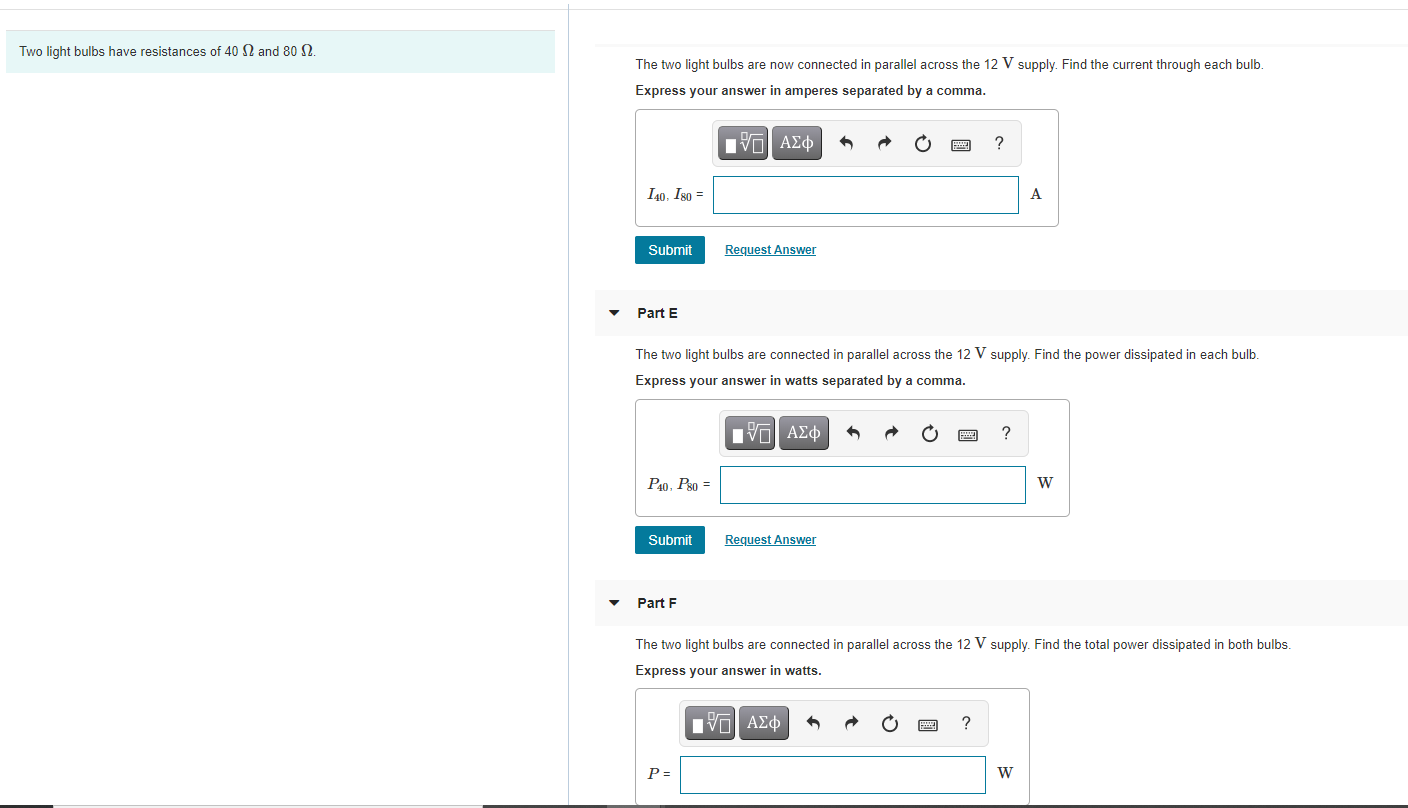Two light bulbs have resistances of 40 2 and 802. The two light bulbs are now connected in parallel across the 12 V supply. Find the current through each bulb. Express your answer in amperes separated by a comma. VASO 140, 180 = A Submit Request Answer Part E The two light bulbs are connected in parallel across the 12 V supply. Find the power dissipated in each bulb. Express your answer in watts separated by a comma. LOAD ?...

• ### Two light bulbs are connected in parallel, and then connected to a battery, as shown in...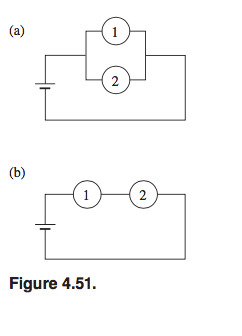Two light bulbs are connected in parallel, and then connected to a battery, as shown in Fig. 4.51(a). You observe that bulb 1 is twice as bright as bulb 2. Assuming that the brightness of a bulb is proportional to the power dissipated in the bulb’s resistor, which bulb’s resistor is larger, and by what factor? The bulbs are now connected in series ,as shown in Fig.4.51(b). Which bulb is brighter, and by what factor? How bright is each bulb...

• ### several 60 w light bulbs are connected in parallel to a 120 v source. the circuit...

several 60 w light bulbs are connected in parallel to a 120 v source. the circuit has a circuit breaker cb that is placed right after the battery that interrupts the current through itself whenever that current exceeds 9 A. Calculate the largest number of bulbs that can glow together.

• ### Three 70.0-W, 130-V lightbulbs are connected across a ΔV = 130-V power source, as shown in...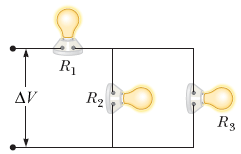Three 70.0-W, 130-V lightbulbs are connected across a ΔV = 130-V power source, as shown in the figure below. Find the total power delivered to the three bulbs and the potential difference across each. Assume the resistance of each bulb is constant (even though, in reality, the resistance increases markedly with current). An open circuit begins at a terminal with a wire extending horizontally rightwards until it reaches a lightbulb R1. After the light bulb, the wire splits downwards into...

• ### 5. Are light bulbs brighter when they're connected in series or in parallel? 6. Consider a...

5. Are light bulbs brighter when they're connected in series or in parallel? 6. Consider a whole circuit. When the power supply is set to 12V, does the power supply provide more current to the whole circuit when it consists of 3 light bulbs connected in parallel or 3 light bulbs connected in series?

• ### Two light bulbs have resistances of 40 2 and 80 22. Part B The two light...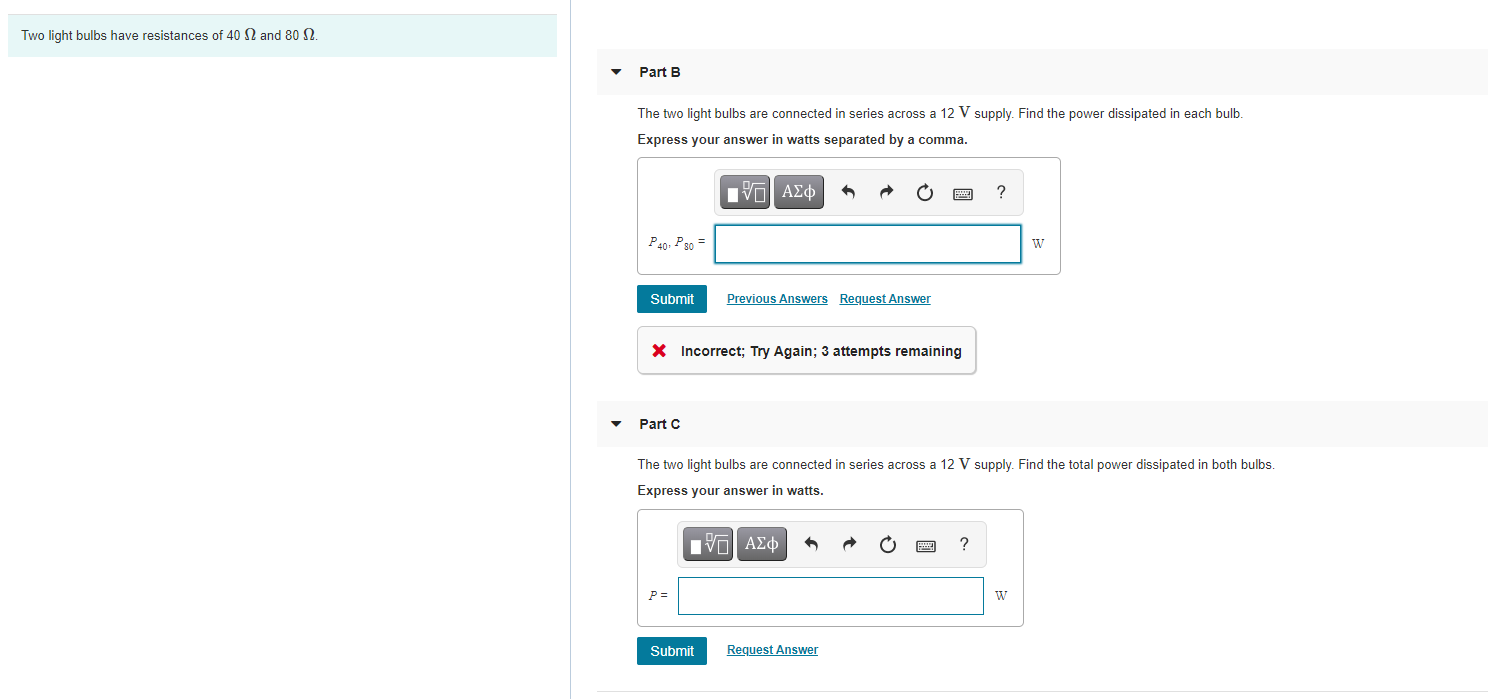Two light bulbs have resistances of 40 2 and 80 22. Part B The two light bulbs are connected in series across a 12 V supply. Find the power dissipated in each bulb. Express your answer in watts separated by a comma. ΙΕΙ ΑΣΦ ? P40, P80 = Submit Previous Answers Request Answer X Incorrect; Try Again; 3 attempts remaining Part C The two light bulbs are connected in series across a 12 V supply. Find the total power dissipated...

• ### a.When two resistors RA and RB are combined in series and connected to a battery it...

a.When two resistors RA and RB are combined in series and connected to a battery it is observed that the ratio of the power dissipated in RA to that dissipated in RB is equal to 10. If this arrangement is dismantled and the same two resistors connected in parallel to the same battery, what is the ratio of the power dissipated in RA to that dissipated in RB in this new circuit? b.How much energy is converted by a 12...

• ### Three identical light bulbs R. R, and R, are connected across a 120V power source as...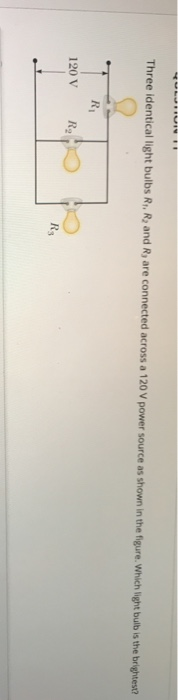Three identical light bulbs R. R, and R, are connected across a 120V power source as shown in the figure. Which light bulb is the brightest? R 120 V Rg R

• ### Two light bulbs rated at 60W/120V are connected in parallel. What is the total power used...

Two light bulbs rated at 60W/120V are connected in parallel. What is the total power used by the bulb ?

• ### Two light bulbs have resistances of 400 Ω and 800 Ω The two light bulbs are...

Two light bulbs have resistances of 400 Ω and 800 Ω The two light bulbs are connected in series across a 120- V line. Find the current through each bulb. Enter your answers numerically separated by a comma. I 400, I 800 =______A Part B Find the power dissipated in each bulb. P 400, P 800 =________W Part C Find the total power dissipated in both bulbs. P =______W Can someone explain how to go about this question? Thank you.